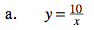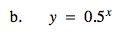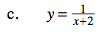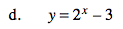### Home > PC > Chapter 8 > Lesson 8.1.1 > Problem8-15

8-15.
1. Find the equations of the asymptotes for each of the following functions. Homework Help ✎

1. y =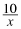2. y = 0.5x

3. y =4. y = 2x − 3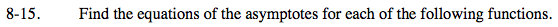Vertical asymptotes occur when there is division by zero.
To identify horizontal asymptotes, think about what happens to the function as x becomes a very large number.